# How to Use VLOOKUP and the True and False Value Correctly in Excel 2007 and 2010

Robbie mostly writes about Skyrim but also occasionally sheds light on the oddities of Microsoft applications such as Excel and Outlook.

Welcome to my second article on the VLOOKUP function in Excel. In today’s article, I am looking at how the TRUE and FALSE values work within the VLOOKUP function and how to avoid getting an incorrect value returned when using the TRUE flag in particular. VLOOKUP allows you to find a value within a range of cells elsewhere in your workbook and return information about it. To use an example:

I have a music store and use Excel to store my customer orders. I want Excel to automatically tell me the cost of a customers order. So for example, a customer orders Green by R.E.M. on CD. I enter the order into my spreadsheet and Excel checks my catalogue and returns with the cost of the album.

I have an article that covers VLOOKUP in further detail using my music store example. This article can be found here.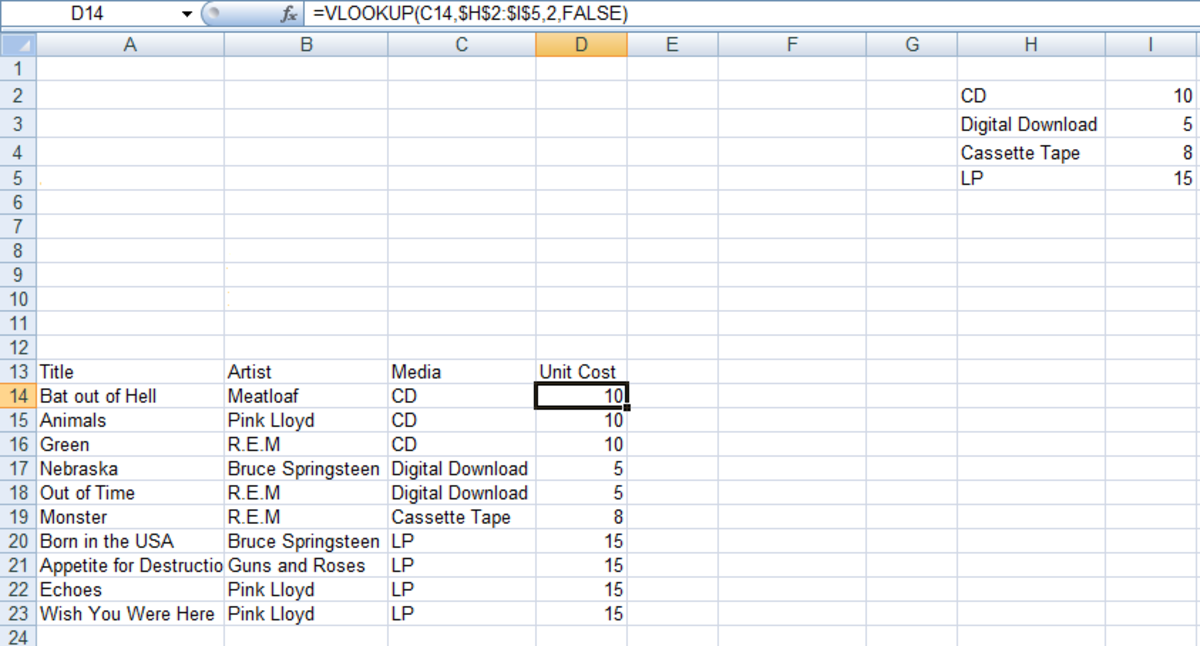Example of how to use VLOOKUP to return information about an item you are interested in (in this example an item's cost) using Excel 2007 and Excel 2010.http://robbiecwilson.hubpages.com/

## What the TRUE and FALSE Values Do Within the VLOOKUP Function in Excel 2007 and 2010

The TRUE and FALSE values are together known as the Range_lookup value within the VLOOKUP function. They allow you to tell Excel if it should be looking for an exact match or an approximate match compared to the contents of the cell you are interested in. The way they work is summarised in the table below

ValueExcel looks forExcel returns

TRUE

An exact, or approximate match

If Excel is unable to find an exact match, the next value that is less than the value you are interested in is returned

FALSE

An exact match

If Excel is unable to find a match, it returns #N/A

As you can see from the above table, Excel will return an approximate value if you choose TRUE and the exact value is not within the range you specify.

## How Using the TRUE Value Can Return Incorrect Answers When Using VLOOKUP in Excel 2007 and 2010

To illustrate the problems that can occur when the TRUE value is not used correctly, we will work through an example.

In this example, I have a class of students entering university. I have all of their University Entrance (UE) results and want to be able to type in their names and have Excel fetch their UE results automatically.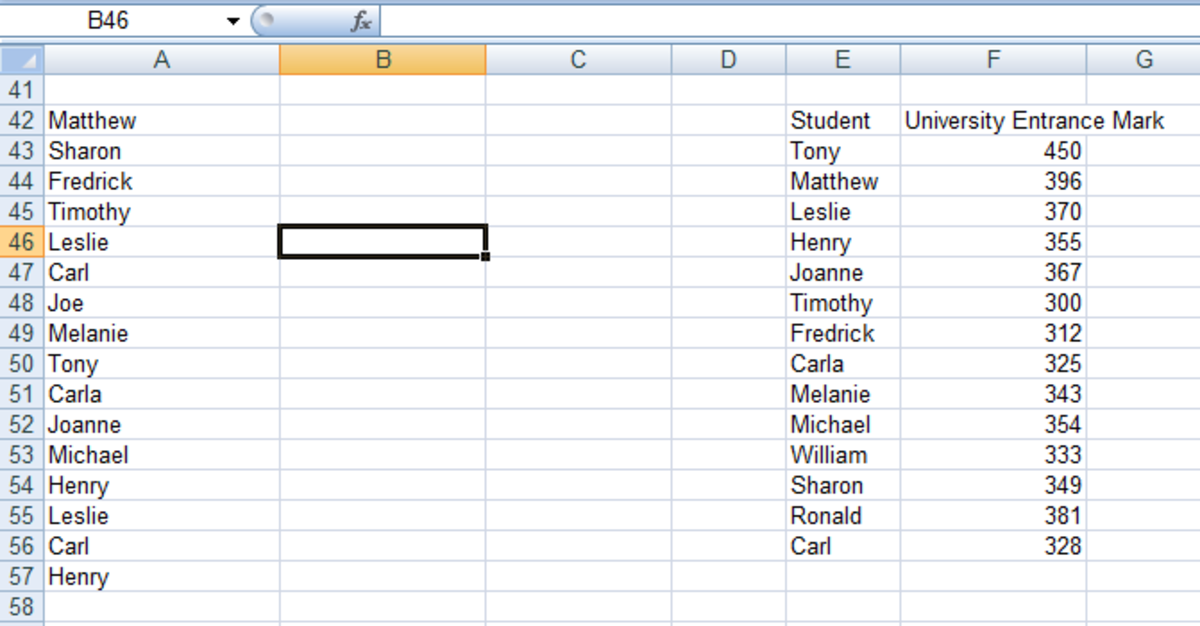Data that will be used to illustrate how the TRUE value in VLOOKUP can return incorrect results in Excel 2007 and Excel 2010.http://robbiecwilson.hubpages.com/

In the figure above, you can see the list of the students and their grades in the cells E43:F56 in my workbook. Excel will check this range for a name that matches the name in the cell I specify (A42 for the first student Matthew). To achieve this, I will use the following formula:

=VLOOKUP(A42,\$E\$43:\$F\$56,2,TRUE)

The formula is made up of the following components:

1. The target cell you are asking Excel to compare A42.
2. The range of cells you are asking Excel to compare the target against \$E\$43:\$F\$56.
3. The number of columns in the range 2.
4. Whether Excel is looking for an approximate or exact match TRUE.

Excel comes back with some rather curious results as shown below.

Scroll to Continue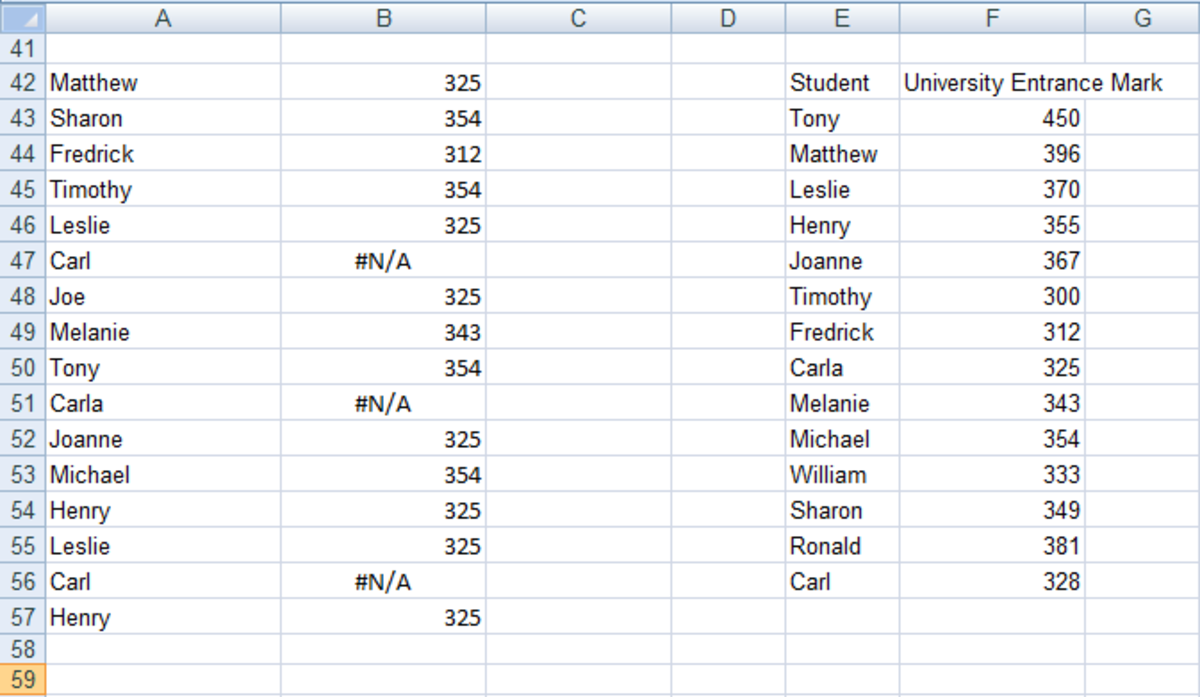Example of VLOOKUP returning incorrect results with the TRUE value in Excel 2007 and Excel 2010.http://robbiecwilson.hubpages.com/

If you look at the results, Excel has indeed thrown up some very strange results. I have coloured the results as green if correct and red if incorrect.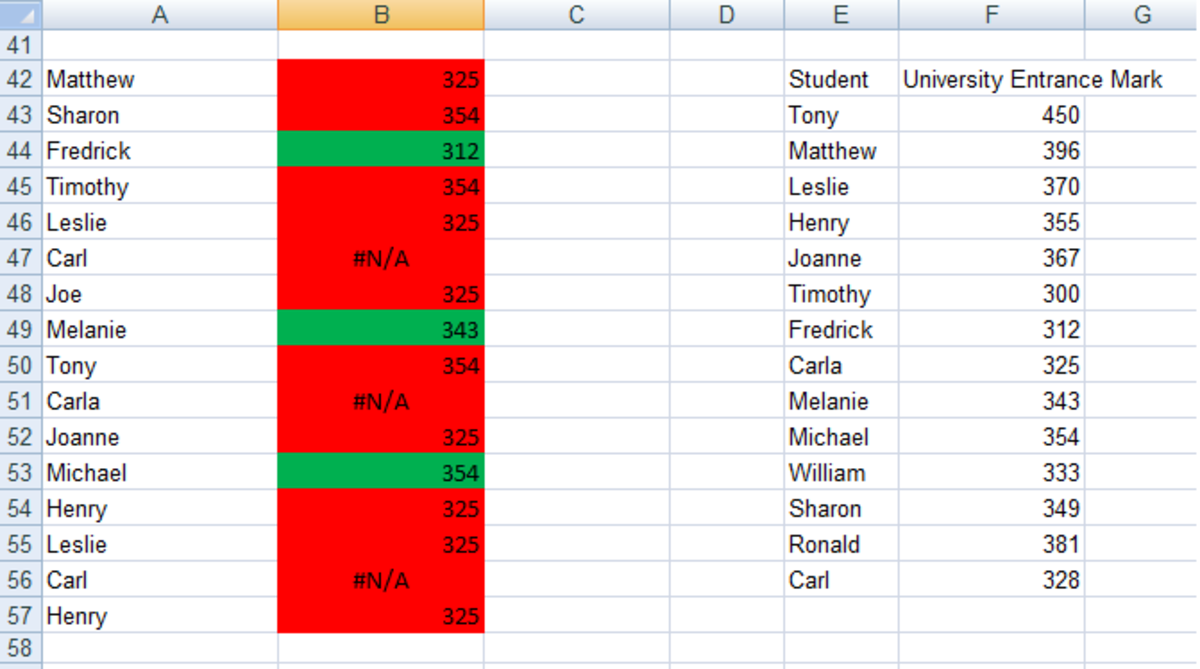Example of VLOOKUP returning incorrect (coloured red) results with the TRUE value in Excel 2007 and Excel 2010.http://robbiecwilson.hubpages.com/

Of those incorrect results, Joe is not even in the list of students, although he was given a score of 325! Carl and Carla both got #N/A rather than a number and Excel got 3/16 correct.

## How to Use VLOOKUP With TRUE and Obtain Correct Results in Excel 2007 and 2010

The secret to using VLOOKUP with TRUE and having it return 100% correct results lies with the reference date you ask Excel to compare the cell you are interested with.

If you sort the data then it will return correct results. To do this:

• Select the first row of the reference data (in my example above, it is E43: E55).
• Click on the Sort A to Z button on the Data tab in the Sort & Filter group.
• Select Expand the selection so that it sorts F43:F55 so that the student’s names match their results once sorted.
• Click OK.

The VLOOKUP results will update automatically. I have again coloured the cells where Excel returns the correct result as green and incorrect results as red in the figure below.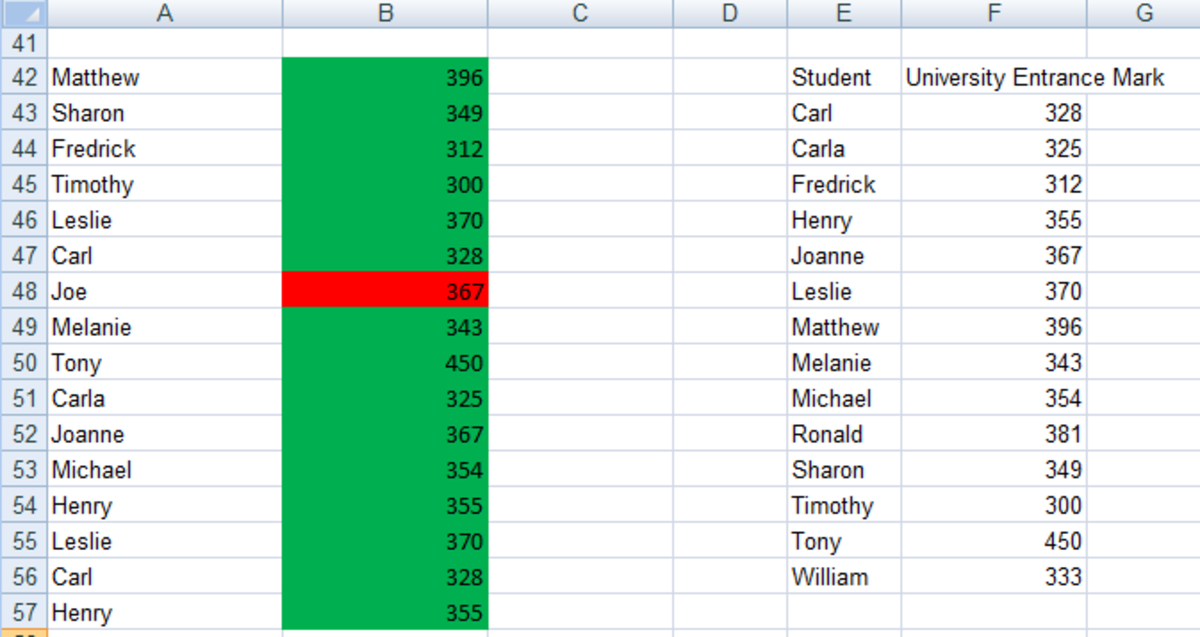VLOOKUP results with TRUE after the data has been sorted in Excel 2007 and Excel 2010.http://robbiecwilson.hubpages.com/

This time, Excel got all the results right except that it gave the mysterious Joe a result of 367 despite not existing. The eagle eyed amongst you will notice that he was given Joanne’s result. This in fact is not an incorrect result at all as we were using TRUE, which returns the exact result OR the nearest result it can find (the next largest when working with numbers). This illustrates the importance of using TRUE and FALSE correctly.

## Using VLOOKUP With the FALSE Value in Excel 2007 and 2010

The FALSE value returns either the exact match or an error if it is unable to find an exact match so whether the reference data is sorted or not, it comes back with 15 correct answers out of 16 and a #N/A for Joe as Joe does not exist in the reference data.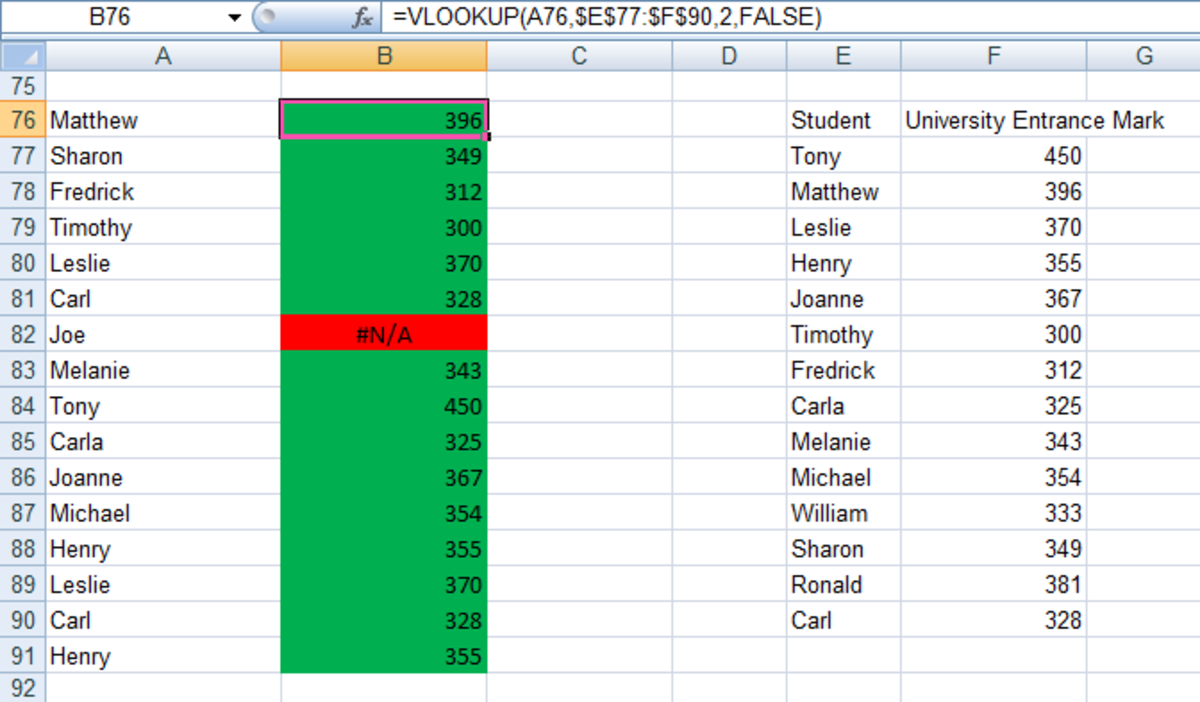VLOOKUP results using the FALSE value to return exact matches in Excel 2007 and Excel 2010.http://robbiecwilson.hubpages.com/

## Other Instances Where VLOOKUP Can Return Incorrect Results in Excel 2007 and 2010

There are two other instances where VLOOKUP can fail to return accurate results.

The first is especially important if you are importing or copying your reference data (the range of cells Excel will compare the target cell with).

• If your reference data has leading or trailing spaces or non-printable characters this can lead to unpredictable results. Trailing spaces are particularly difficult to spot in your workbook, so use the CLEAN function to remove any unprintable characters or TRIM to delete any spaces added to the cell (except for any single spaces between words).

I have an article that covers using both the TRIM and CLEAN functions, which can be found here.

• The second instance is when you have dates or numbers stored as text. This is also likely to result in Excel throwing back unexpected results.

## Conclusion

The VLOOKUP function is an immensely powerful and very useful function in Excel 2007 and 2010. In today’s article:

• We have looked at how the TRUE value looks at your reference data in your workbook and returns either the exact result, or an approximation.
• We then investigated how using the TRUE value can lead to Excel returning incorrect results and how to avoid this by sorting the reference data.
• Finally, we examined other instances where VLOOKUP can return incorrect data when the data has trailing or leading spaces or unprintable characters and how to fix this using the TRIM and CLEAN functions as well as ensuring the numbers and dates are not stored as text.

When using the TRUE value with VLOOKUP, it is essential to sort the data to ensure that Excel returns accurate data. The FALSE value does not need the data to be sorted as it is looking only for exact matches. Whichever value you choose to use, it is important to understand how both work and how to ensure that Excel returns accurate answers for you.

I do hope that you have found this article useful and informative and that you are now able to use VLOOKUP so that it always returns the correct result. Many thanks for reading and feel free to leave any comments you may have below.

viraja on February 08, 2019:

Harry on August 11, 2018:

Sir, is it possible to use true and false (range_lookup) in the same vlookup formula, if so will we be able to derive correct answer?

Robbie C Wilson (author) on March 10, 2015:

Hi Doris,

Thanks for your kind comment, I am glad that you found my Hub useful and informative :)

Mohammad Tanvir Ibne Amin from Dhaka on December 05, 2014:

Its very difficult to work with MSExcel. But the way you described in the article is very useful. Great hub.